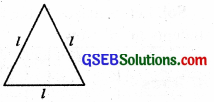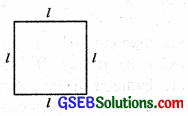# GSEB Class 6 Maths Notes Chapter 11 Algebra

This GSEB Class 6 Maths Notes Chapter 11 Algebra covers all the important topics and concepts as mentioned in the chapter.

## Algebra Class 6 GSEB Notes

Introduction:
Algebra is a branch of mathematics in which symbols (usually Letters) represent unknown numbers in mathematical equations. Algebra allows the basic operations of arithmetic, such as addition, subtraction and multiplication, to be performed without using specific numbers.

We use algebra in everyday life. For example, to calculate how much flour you need to bake a certain number of cookies or how long it will take to travel by car at a certain speed to cover a certain distance.

Main Features of Algebra

• The main feature of Algebra is the use of letters. The letters will allow us to write rules and formulas in a general way. By using letters, we can talk about any number and not just a particular number.
• These letters in algebra may represent unknown numbers. These are called literals or variables. The letters like a, b,c, ….p,q,r,…. x, y, z are called literals or variables. By learning methods of determining variables, we develop powerful rules for solving puzzles and many problems from our daily life.
• Since letters stand for numbers, operations can be performed on them as on numbers. This leads to the study of algebraic expressions and their properties.

Variables and Constants:
Constant:
A quantity which takes a fixed numerical value is called a constant.
Example : 5, 7, $$\frac{-3}{2}$$ are constants.

Variable:
A quantity which takes on various numerical values is called a variable.
Example : The literal numbers A, x, a, P, S etc. are variables.

Use of the Variables in Common Rules
Rules From Geometry.
1. Perimeter of an equilateral triangle:
We know that the perimeter of a triangle is the sum of the length of its sides. Here, let the side of the triangle be T then,
Perimeter = l + l + l = 3l.Thus we get the rule for the perimeter of an equilateral triangle. Here 3 is a constant and l is a variable. The use of the variable l allows us to write the general rule which is easy to remember.

2. Perimeter of square:
A square has 4 sides and they all are equal. Let the side of square be T∴ The perimeter a square = sum of the length of the 4 sides of squares = l + l + l + l = 4l
Thus, we get the rule for the perimeter of square.

3. Perimeter of a rectangle:
The Perimeter of a rectangle with length 7’ and breadth ‘b’ is given by P = 2 (l + b).
Here there are two variables ‘l’ and ‘b’ independent of each other.Rules From Arithmetic.
1. Commutative Property:

If the order of numbers in addition is changed, it does not change their sum. If a and b are two variables which can take any numerical value then we have, a + b = b + a
• Multiplication:
If the order of numbers in multiplication is changed, it does not change their product.
a × b = b × a (Commutative property of multiplication)

2. Associative property.

If three numbers can be added in any order, it does not change their sum. Let a, b and c be any three variables representing any three number, then
(a + b) + c = (a + b) + c
• Multiplication:
If three numbers can be multiplied in any order, it does not change their product. a × (b × c) = (a × b) × c

3. Distributive Property:

• Multiplication Over Addition. Let a, b and c be the three variables representing any there numbers, then
a × (b + c) = a × b + a × c
• Multiplication Over Subtraction.
Let a, b and c be the three variables representing three numbers then,
a × (b – c) = a × b – a × c

Algebraic Expressions (Expressions with Variables)
Definition:
A number or a combination of numbers (including literal numbers), using the signs of fundamental operation (+, x, -, ÷), is called an algebraic expression.
Example : $$\frac{5}{7}, \frac{-3}{4}$$, 2x + 3, a – 2b + c, + b3 + c3 + 3abc etc. are all algebraical expression

Practical use of Algebraic Expressions
If we are given a mathematical statement in an ordinary language, we can convert it into an algebraic expression. Some examples are given below :
Statement: Ram is 2 years younger than his sister Kanika.

Algebraic Expression:
Let Kanika’s age be x years then Ram’s age is (x – 2) years.
Here, (x – 2) is an algebraic expression.
Statement: Price of milk per litre is ₹ 2 more than 5 times the price of one kg onion.

Algebraic Expression:
Let the price of the onion be ₹ x per kg. then the price of milk per litre is ₹ (5x + 2). Here (5x + 2) is an algebraic expression.

What is an Equation?
Equation:
A statement of equality which involves one or more literal numbers is called an equation. In other words,
A mathematical Statement that one expression is equal to another, i.e., two quantities are joined by an equal’s sign is called an equation.
Those equations which have one or more variables (unknown values) and their highest power is 1 are called linear equations.
e.g. 3x + 5 = 9, $$\frac{x}{2}$$ + 5 = 3 are the examples of linear equations.Solution or Root of an Equation
A number which when substituted for the variable in the equation makes its L.H.S. equal to R.H.S., is said to satisfy the equation and is called a solution or a root of the equation.

To solve an equation or to find the solution (root) of an equation, we can follow the following methods :
1. Trial and Error Method:
In this method, we try different values for the variable (unknown number) to make both sides of an equation equal. When we get a particular value of the variable which makes L.H.S. equal to R.H.S. This particular value is said to be the root of the equation.

2. Systematic Method:
An equation behaves like a weighing balance. Both sides of equation are balanced in the same manner as the scales of a balance. When the weights in both sides are equal, the weighing balance is balanced. We can add equal weights to both sides or remove equal weights from both sides then also the two sides will be in balance.

Here we have four rules (axioms) for balancing linear equations.

• Rule 1. If we add the same number (quantity) on both sides of an equation, the equality holds true.
• Rule 2. If we subtract same number from both sides of an equation, the equality holds true.
• Rule 3. If we multiply both sides of an equation by the same number, the equality holds true.
• Rule 4. If we divide both sides of an equation by the same number, the equality holds true.

3. Method of Transposition a number:
Transposing a number (i. e. changing the side of the number) is the same as adding or subtracting the number, multiplying or dividing by the number to both sides of the equation; we change the sign + into and vice-versa, ‘x’ into ‘÷’ and vice-versa.